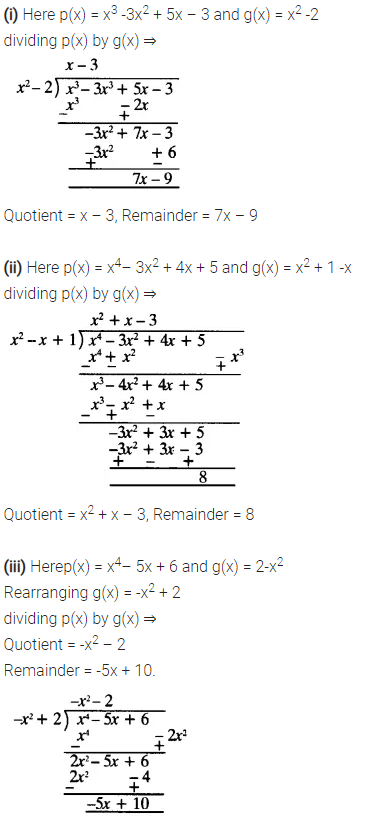ncert textbook

# NCERT Solutions For Class 10 Maths Chapter 2 Ex 2.3

Get Free NCERT Solutions for Class 10 Maths Chapter 2 Ex 2.3 PDF. Polynomials Class 10 Maths NCERT Solutions are extremely helpful while doing homework. Exercise 2.3 Class 10 Maths NCERT Solutions were prepared by Experienced ncert-books.inTeachers. Detailed answers of all the questions in Chapter 2 maths class 10 Polynomials Exercise 2.3 provided in NCERT TextBook.

Topics and Sub Topics in Class 10 Maths Chapter 2 Polynomials:

 Section Name Topic Name 2 Polynomials 2.1 Introduction 2.2 Geometrical Meaning Of The Zeroes Of A Polynomial 2.3 Relationship Between Zeroes And Coefficients Of A Polynomial 2.4 Division Algorithm For Polynomials 2.5 Polynomials 2.6 Summary

You can also download the free PDF of  Ex 2.3 Class 10 Polynomials NCERT Solutions or save the solution images and take the print out to keep it handy for your exam preparation.

 Board CBSE Textbook NCERT Class Class 10 Subject Maths Chapter Chapter 2 Chapter Name Polynomials Exercise Ex 2.3 Number of Questions Solved 5 Category NCERT Solutions

## NCERT Solutions For Class 10 Maths Chapter 2 Ex 2.3

NCERT Solutions for Class 10 Maths Chapter 2 Polynomials Ex 2.3 are part of NCERT Solutions for Class 10 Maths. Here we have given Maths NCERT Solutions Class 10 Chapter 2 Polynomials Exercise 2.3.
Question 1.
Divide the polynomial p(x) by the polynomial g(x) and find the quotient and remainder in each of the following:
(i) p(x) = x3 – 3x2 + 5x – 3, g(x) = x2 – 2
(ii) p(x) = x4 – 3x2 + 4x + 5, g(x) = x2 + 1 – x
(iii) p(x) = x4– 5x + 6, g(x) = 2 – x2
Solution:Question 2.
Check whether the first polynomial is a factor of the second polynomial by dividing the second polynomial by the first polynomial.
(i) t2 – 3, 2t4 + 3t3 – 2t2– 9t – 12
(ii) x2 + 3x + 1, 3x4 + 5x3 – 7x2 + 2x + 2
(iii) x2 + 3x + 1, x5 – 4x+ x2 + 3x + 1
Solution:Question 3.
Obtain all other zeroes of 3x4 + 6x3 – 2x2 – 10x – 5, if two of its zeroes are  and$\sqrt { \frac { 5 }{ 3 } }$and –$\sqrt { \frac { 5 }{ 3 } }$
Solution:Question 4.
On dividing x– 3x2 + x + 2bya polynomial g(x), the quotient and remainder were x – 2 and -2x + 4 respectively. Find g(x).
Solution:Question 5.
Give examples of polynomials p(x), g(x), q(x) and r(x), which satisfy the division algorithm and:
(i) deg p(x) = deg q(x)
(ii) deg q(x) = deg r(x)
(iii) deg r(x) = 0
Solution: